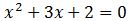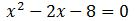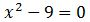# Solving Problems using the Quadratic Formula

Instructions:

Choose an answer and hit 'next'. You will receive your score and answers at the end.

question 1 of 3

### Solve.Create Your Account To Take This Quiz

As a member, you'll also get unlimited access to over 75,000 lessons in math, English, science, history, and more. Plus, get practice tests, quizzes, and personalized coaching to help you succeed.

Try it risk-free for 30 days. Cancel anytime.

### 1. Solve.### 2. Solve.Create your account to access this entire worksheet
A Premium account gives you access to all lesson, practice exams, quizzes & worksheets
Quizzes, practice exams & worksheets
Certificate of Completion
Create an account to get started

This quiz and attached worksheet will help gauge your understanding of solving problems using the quadratic formula. Topics you will need to know to pass the quiz include variables and quadratic equations.

## Quiz & Worksheet Goals

Use these assessment tools to measure your knowledge of:

• Solving equations and variables

## Skills practiced

This worksheet and quiz let you practice the following skills:

• Critical thinking - apply relevant concepts to examine information about solving problems using the quadratic formula in a different light
• Problem solving - use acquired knowledge to solve quadratic formula practice problems
• Interpreting information - verify that you can read information regarding problems using the quadratic formula and interpret it correctly
• Knowledge application - use your knowledge to answer questions about solving problems using the quadratic formula

To learn more about solving problems using the quadratic formula, review the lesson Solving Problems using Quadratic Formula which covers the following objectives:

• Define quadratic formula
• Understanding solutions
Final ExamELM: CSU Math Study Guide
Status: Not Started
Chapter ExamELM Test - Algebra: Polynomials
Status: Not Started

Support